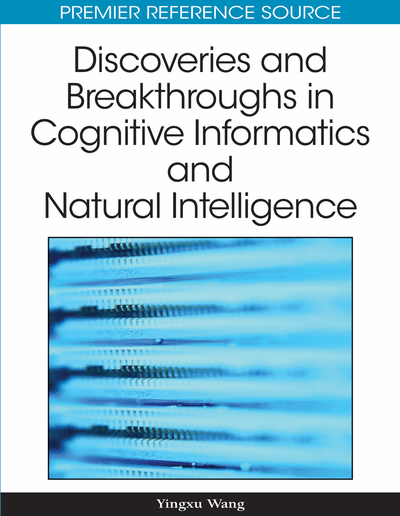# Foundation and Classification of Nonconventional Neural Units and Paradigm of Nonsynaptic Neural Interaction

I. Bukovsky (Czech Technical University, Czech Republic), J. Bila (Czech Technical University, Czech Republic), M. M. Gupta (University of Saskatchewan, Canada), Z. G. Hou (The Chinese Academy of Sciences, P.R. China) and N. Homma (Tohoku University, Japan)
DOI: 10.4018/978-1-60566-902-1.ch027
Available
\$37.50
No Current Special Offers

## Abstract

This chapter introduces basic types of nonconventional neural units and focuses their mathematical notation and classification. Namely, the notation and classification of higher-order nonlinear neural units, time-delay dynamic neural units, and time-delay higher-order nonlinear neural units is introduced. The classification of nonconventional neural units is founded first according to nonlinearity of aggregating function, second according to the dynamic order, third according to time-delay implementation within neural units. Introduction into the simplified parallel of the higher-order nonlinear aggregating function of higher-order neural units revealing both the synaptic and nonsynaptic neural interaction is made; thus, a new parallel between the mathematical notation of nonconventional neural units and the neural signal processing of biological neurons and is drawn. Based on the mathematical notation of neural input inter-correlations of higher-order neural units, it is shown that higher-order polynomial aggregating function of neural inputs can be naturally understood as a single-equation representation consisting of synaptic neural operation plus nonsynaptic neural operation. Thus it unravels new simplified yet universal mathematical insight into understanding the higher computational power of neurons that also conforms to biological neuronal morphology according to nowadays achievements of biomedical sciences.
Chapter Preview
Top

## Introduction

Neural networks are powerful cognitive tools with learning and generalization capability; however, the black-box or sometimes gray-box effect of neural networks is a well known drawback that often prevents researchers from further network analysis and utilization of learned knowledge hidden in the network, e.g. to analyze generalization capabilities of the network for a particular real system. In neural computation oriented literature, the focus is usually put on a neural network as whole and the computational strength is naturally to be induced by neuronal networking. The network complexity and the number of neural parameters should be minimized while its maximum computational power and generalization capability has to be maintained in order to minimize the black-box effect of neural networks. As seen from the multidisciplinary point of view, there are three major attributes that establish complex behavior of systems and that can be used to mathematically describe complex system behavior. These attributes are the nonlinearity, dynamics, and time delays; these attributes also appear in neurons and neural networks. These three attributes can be simultaneously implemented in individual neurons to maximize their individual approximation capability and thus to minimize neural network complexity and to maximize its cognitive capabilities. In this paper we propose (or highlight) new emerging notation and classification of neural units that emerges from neural network discipline as well as from concepts common to various fields of science. We also propose a discussion on a plausible neurological aspect of a full polynomial notation of neural aggregation function in the next subsection.

An important nonlinearity attribute in neural network was significantly proposed in neural networks using higher–order polynomials as neural activation function (Ivakchnenko, 1971; Ivakchenko & Iba, 2003). An exhaustive survey has been made by Duch & Jankowski (1999) where various neural activation functions and neural output functions are investigated and summarized. For simple demonstration of our proposed classification approach and also for their universal approximating and correlating capabilities, only the polynomials are considered as neural input aggregating functions (activation functions) optionally including neural states (in case of dynamic neural units). An alternative concept of higher-order nonlinear neural units (HONNU) was recently published by M.M. Gupta et al. (2003, pp.279-286). Therefore, the conception and terminology used by M.M. Gupta et al. in their book on neural networks (Gupta et al., 2003) is followed in this work. Typical representatives of HONNU are namely the quadratic neural unit (QNU) and the cubic neural unit (CNU) that were consequently investigated. The results were published by the research group of M.M. Gupta (Redlapalli et al., 2003), where a higher-order polynomial nonlinear aggregating function has been originally understood as a merely synaptic neural operation, i.e., as a synaptic neural input preprocessor. More recently, an application of HONNU to robot image processing was published by Hou et al. (2007) with similar interpretation of a synaptic neural operation of HONNU. Except the proposed classification of nonconventional neural units in this paper, then next section shows that polynomial aggregating functions of neural inputs includes in principle also nonsynaptic and possibly somatic neural transmissions.

## Complete Chapter List

Search this Book:
Reset